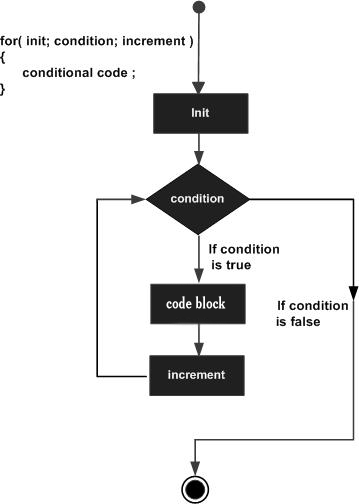# D Programming - for Loop

A for loop is a repetition control structure that allows you to efficiently write a loop that needs to execute a specific number of times.

## Syntax

The syntax of a for loop in D programming language is −

```for ( init; condition; increment ) {
statement(s);
}
```

Here is the flow of control during a for loop −

• The init step is executed first, and only once. This step allows you to declare and initialize any loop control variables. You are not required to put a statement here, as long as a semicolon appears.

• Next, the condition is evaluated. If it is true, the body of the loop is executed. If it is false, the body of the loop does not execute and flow of control jumps to the next statement just after the for loop.

• After the body of the for loop executes, the flow of control jumps back up to the increment statement. This statement allows you to update any loop control variables. This statement can be left blank, as long as a semicolon appears after the condition.

• The condition is now evaluated again. If it is true, the loop executes and the process repeats itself (body of loop, then increment step, and then again condition). After the condition becomes false, the for loop terminates.

## Flow Diagram## Example

```import std.stdio;

int main () {
/* for loop execution */
for( int a = 10; a < 20; a = a + 1 ) {
writefln("value of a: %d", a);
}

return 0;
}
```

When the above code is compiled and executed, it produces the following result −

```value of a: 10
value of a: 11
value of a: 12
value of a: 13
value of a: 14
value of a: 15
value of a: 16
value of a: 17
value of a: 18
value of a: 19
```
d_programming_loops.htm